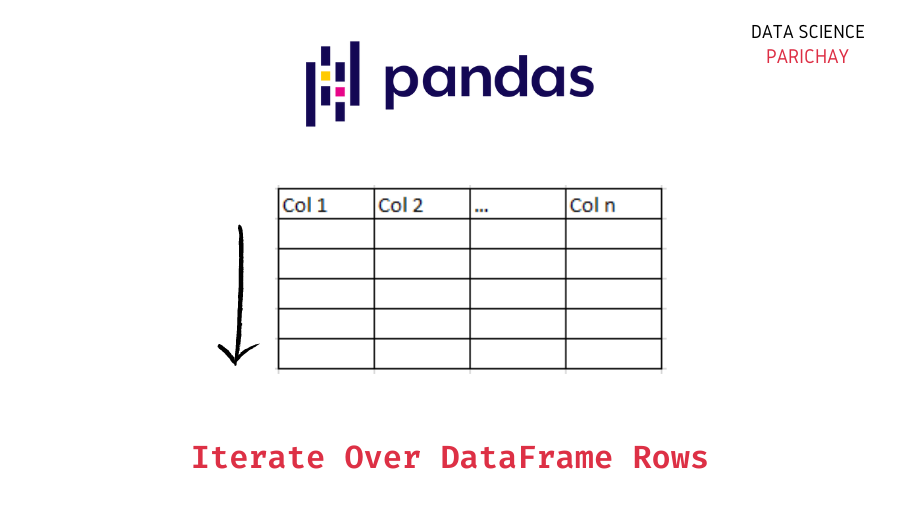# Pandas – Iterate over Rows of a Dataframe

Pandas dataframes are very useful for accessing and manipulating tabular data in Python. It can be handy to know how to iterate over the rows of a Pandas dataframe. In this tutorial, we’ll look at some of the different methods using which we can iterate or loop over the individual rows of a dataframe in pandas.

In Pandas, the iterrows() function is generally used to iterate over the rows of a dataframe as (index, Series) tuple pairs. You can also use the itertuples() function which iterates over the rows as named tuples.

Let’s look at some examples of how to iterate over a dataframe’s rows.

First, let’s create a sample dataframe which we’ll be using throughout this tutorial. You can follow along by using the code in this tutorial and implementing it in the environment of your choice.

```import pandas as pd

# raw data
data = {
"Name": ["Nick", "Kate", "Rohan", "Sam", "Emma"],
"Age": [23, 24, 26, 21, 22],
"Country": ["India", "USA", "UK", "Canada", "Germany"],
}

# create a dataframe
df = pd.DataFrame(data, columns=["Name", "Age", "Country"])

# display the dataframe
print(df)```

Output:

```    Name  Age  Country
0   Nick   23    India
1   Kate   24      USA
2  Rohan   26       UK
4   Emma   22  Germany```

The dataframe `df` contains the information regarding the `Name`, `Age`, and `Country` of five people with each represented by a row in the dataframe.

The Pandas `iterrows()` function is used to iterate over dataframe rows as (index, Series) tuple pairs. Using it we can access the index and content of each row. The content of a row is represented as a Pandas Series.

Since iterrows returns an iterator we use the `next()` function to get an individual row. We can see below that it is returned as an (index, Series) tuple.

📚 Data Science Programs By Skill Level

Introductory

Intermediate ⭐⭐⭐

🔎 Find Data Science Programs 👨‍💻 111,889 already enrolled

Disclaimer: Data Science Parichay is reader supported. When you purchase a course through a link on this site, we may earn a small commission at no additional cost to you. Earned commissions help support this website and its team of writers.

`next(df.iterrows())`

Output:

```(0,
Name        Nick
Age           23
Country    India
Name: 0, dtype: object)```

#### Iterating over all rows using iterrows()

Generally, `iterrows()` is used along with `for` to loop through the rows. The contents of a row are returned as a Series and hence can be accessed by their column name as shown below –

```# iterate over all the rows
for index, row in df.iterrows():
print("Index:", index)
print("Name:", row["Name"])```

Output:

```Index: 0
Name: Nick
Index: 1
Name: Kate
Index: 2
Name: Rohan
Index: 3
Name: Sam
Index: 4
Name: Emma```

The Pandas documentation mentions that “You should never modify something you are iterating over. This is not guaranteed to work in all cases. Depending on the data types, the iterator returns a copy and not a view, and writing to it will have no effect.” See the example below –

```# print the dataframe
print("Dataframe before iterrows:\n", df)

for index, row in df.iterrows():
# trying to rename each Name to ABC
row["Name"] = "ABC"

# print the dataframe
print("\nDataframe after iterrows:\n", df)```

Output:

```Dataframe before iterrows:
Name  Age  Country
0   Nick   23    India
1   Kate   24      USA
2  Rohan   26       UK
4   Emma   22  Germany

Dataframe after iterrows:
Name  Age  Country
0   Nick   23    India
1   Kate   24      USA
2  Rohan   26       UK
4   Emma   22  Germany```

In the above example, we see that trying to modify the dataframe `df` by changing the row returned by `iterrows()` did not have any effect on the dataframe `df`. This is because the iterator returns a copy and not a view and writing to it has no effect on the original dataframe.

The Pandas `itertuples()` function is used to iterate over dataframe rows as named tuples.

`next(df.itertuples())`

Output:

`Pandas(Index=0, Name='Nick', Age=23, Country='India')`

You can also remove the index and give custom name to the rows returned by itertuples()

`next(df.itertuples(index=False, name="Person"))`

Output:

`Person(Name='Nick', Age=23, Country='India')`

Like dictionaries, named tuples contain keys that are mapped to some values. There are a number of ways you can access the values of a named tuple. See the example below –

```# get the values for a row
for row in df.itertuples():
print(row)
# using index
print("Name:", row)
# using the key
print("Age:", row.Age)
# using getattr() function
print("Country:", getattr(row, "Country"))```

Output:

```Pandas(Index=0, Name='Nick', Age=23, Country='India')
Name: Nick
Age: 23
Country: India
Pandas(Index=1, Name='Kate', Age=24, Country='USA')
Name: Kate
Age: 24
Country: USA
Pandas(Index=2, Name='Rohan', Age=26, Country='UK')
Name: Rohan
Age: 26
Country: UK
Name: Sam
Age: 21
Pandas(Index=4, Name='Emma', Age=22, Country='Germany')
Name: Emma
Age: 22
Country: Germany
```

With this, we come to the end of this tutorial. The code examples and results presented in this tutorial have been implemented in a Jupyter Notebook with a python (version 3.8.3) kernel having pandas version 1.0.5

You might also be interested in –

•